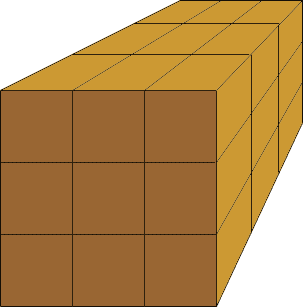Quandaries and Queries Name: neisha Who is asking: Student Level of the question: All Question: Hi, I am trying to figure out how many cubic feet are in 8 yards. I am trying to pour a concrete slab. The width is 24 ft, lenth is 30 ft, and it is 4 inches thick Hi Neisha, A box that is one yard long, one wide and one yard high holds one cubic yard. Since one yard is three feet you can pack your one cubic foot box with 333 = 27 boxes that are one foot long by one foot wide by one foot high.Thus there are 27 cubic feet in one cubic yard. Hence, in eight cubic yards there are 278 = 216 cubic feet. Penny